# Topological ring

(diff) ← Older revision | Latest revision (diff) | Newer revision → (diff)

A ringthat is a topological space, such that the mappings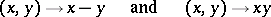are continuous. A topological ringis called separated if it is separated as a topological space (cf. Separation axiom). In this caseis a Hausdorff space. Any subringof a topological ring, and also the quotient ring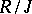by an ideal, is a topological ring. Ifis separated and the idealis closed, thenis a separated topological ring. The closure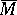of a subringinis also a topological ring. A direct product of topological rings is a topological ring in a natural way.

A homomorphism of topological rings is a ring homomorphism which is also a continuous mapping. If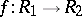is such a homomorphism, whereis moreover an epimorphism and an open mapping, then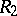is isomorphic as a topological ring to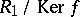. Banach algebras are an example of topological rings. An important type of topological ring is defined by the property that it has a fundamental system of neighbourhoods of zero consisting of some set of ideals. For example, to any ideal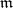in a commutative ringone can associate the-adic topology, in which the setsfor all natural numbersform a fundamental system of neighbourhoods of zero. This topology is separated if the conditionis satisfied.

For a topological ringone can define its completion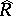, which is a complete topological ring, and a separated topological ringcan be imbedded as an everywhere-dense subset in, which is also separated in this case. The additive group of the ringcoincides with the completion of the additive group of, as an Abelian topological group.

How to Cite This Entry:
Topological ring. Encyclopedia of Mathematics. URL: http://encyclopediaofmath.org/index.php?title=Topological_ring&oldid=17173
This article was adapted from an original article by L.V. Kuz'min (originator), which appeared in Encyclopedia of Mathematics - ISBN 1402006098. See original article